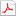검색어 입력폼

반도체 소자공학 pierret 12장

저작시기 2012.12 |등록일 2017.04.03어도비 PDF (pdf) | 15페이지 | 가격 300원

* 본 문서는 배포용으로 복사 및 편집이 불가합니다.

없음

본문내용

(a) Under the quasistatic assumption the carriers and hence the device under analysis are assumed to respond to a time-vaying signal as if it were a d.c. bias. In the derivation of the generalized two-port model, one specifically equates the total time-varying terminal currents(iB, iC) to the d.c. currents that would exist under equivalent viasing conditions.

없음